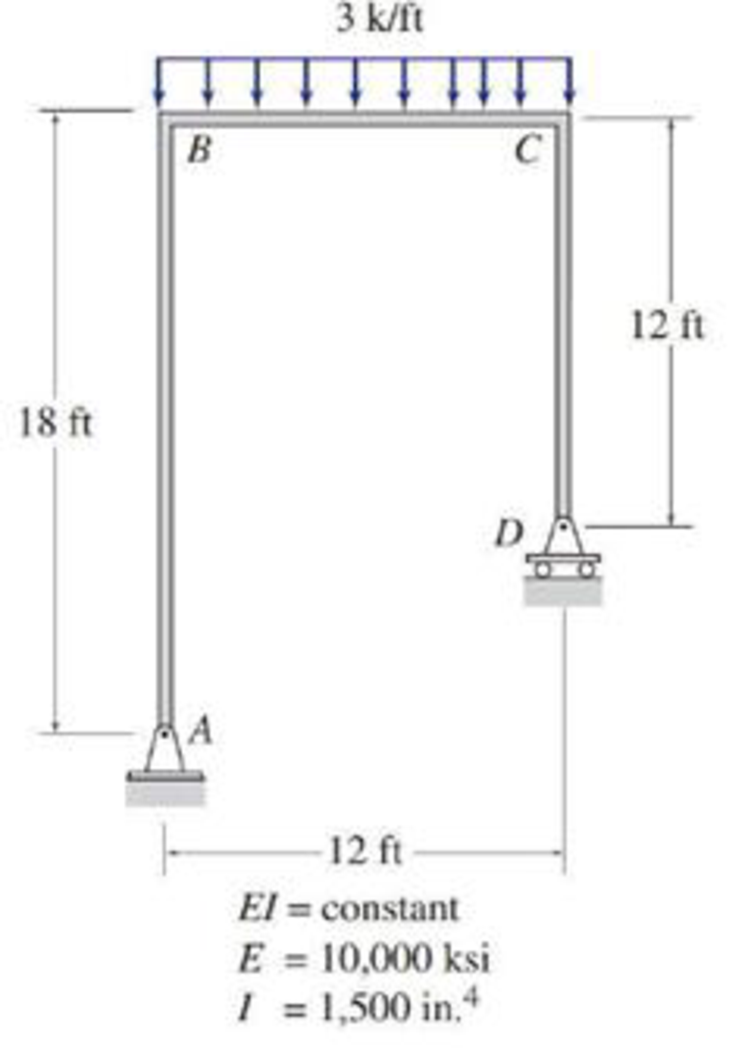# 7.45 and 7.46 Use the virtual work method to determine the horizontal deflection at joint C of the frame shown. FIG. P7.46, P7.69

#### Solutions

Chapter
Section
Chapter 7, Problem 46P
Textbook Problem
221 views

## 7.45 and 7.46 Use the virtual work method to determine the horizontal deflection at joint C of the frame shown.FIG. P7.46, P7.69

To determine

Find the horizontal deflection at joint C of the frame using virtual work method.

### Explanation of Solution

Given information:

The frame is given in the Figure.

The value of E is 10,000 ksi and I is 1,500in.4.

Apply the sign conventions for calculating reactions, forces and moments using the three equations of equilibrium as shown below.

• For summation of forces along x-direction is equal to zero (Fx=0), consider the forces acting towards right side as positive (+) and the forces acting towards left side as negative ().
• For summation of forces along y-direction is equal to zero (Fy=0), consider the upward force as positive (+) and the downward force as negative ().
• For summation of moment about a point is equal to zero (Matapoint=0), consider the clockwise moment as negative and the counter clockwise moment as positive.

Calculation:

Consider the real system.

Draw a diagram showing all the given real loads acting on it.

Let the bending moment due to real load be M.

Sketch the real system of the frame as shown in Figure 1.

Find the reactions at the supports A and D:

Summation of moments about A is equal to 0.

MA=0Dy(12)3(12)(122)=0Dy=18k

Summation of forces along y-direction is equal to 0.

+Fy=0Ay+Dy3(12)=0Ay+183(12)=0Ay=18k

Summation of forces along x-direction is equal to 0.

+Fx=0Ax=0

Consider the virtual system.

Draw a diagram of frame without the given real loads. For horizontal deflection apply unit load at the joint in the horizontal direction.

Let the bending moment due to virtual load be Mv.

Sketch the virtual system of the frame with unit load at joint C as shown in Figure 2.

Find the reactions at the supports A and D:

Summation of moments about A is equal to 0.

MA=0Dy(12)1(18)=0Dy=1.5k

Summation of forces along y-direction is equal to 0.

+Fy=0Ay+Dy=0Ay+1.5=0Ay=1.5k

Summation of forces along x-direction is equal to 0.

+Fx=0Ax+1=0Ax=1k

Find the moment at distance x for the real frame:

M=18x3×x×x2=18x1

### Still sussing out bartleby?

Check out a sample textbook solution.

See a sample solution

#### The Solution to Your Study Problems

Bartleby provides explanations to thousands of textbook problems written by our experts, many with advanced degrees!

Get Started

Find more solutions based on key concepts
Calculate the amount of natural gas that you need to burn to heat twenty gallons of water from room temperature...

Engineering Fundamentals: An Introduction to Engineering (MindTap Course List)

What is a rotary axis?

Precision Machining Technology (MindTap Course List)

How would you explode DFDs?

Systems Analysis and Design (Shelly Cashman Series) (MindTap Course List)

What are some practical applications of virtual reality? (88)

Enhanced Discovering Computers 2017 (Shelly Cashman Series) (MindTap Course List)

If your motherboard supports ECC DDR3 memory, can you substitute non-ECC DDR3 memory?

A+ Guide to Hardware (Standalone Book) (MindTap Course List)

What arc problems can occur in deep or narrow weld joints?

Welding: Principles and Applications (MindTap Course List)